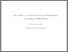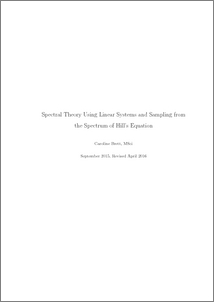# Spectral theory using linear systems and sampling from the spectrum of Hill's equation

Brett, Caroline (2015) Spectral theory using linear systems and sampling from the spectrum of Hill's equation. PhD thesis, UNSPECIFIED.Preview
PDF (2016brettphd)
2016brettphd.pdf - Published Version

## Abstract

This thesis, entitled Spectral Theory Using Linear Systems and Sampling from the Spectrum of Hill’s Equation is submitted by Caroline Brett, Master of Science for the degree of Doctor of Philosophy, September 2015. It uses linear systems to solve various problems connected with Hill’s equation, −f + qf = λf for q ∈ C2, real-valued and π-periodic. Introducing a new operator, Rx constructed from a linear system, (−A, B, C) allows us to solve Hill’s equation and the inverse spectral problem. We use Rx to construct a function, T(x, y) that satisfies a Gelfand–Levitan integral equation and then derive a PDE for T(x, y). Solving this PDE recovers q. Extending Hill’s work in , we show that there exist Hilbert–Schmidt operators, Rp and Rc analogous to Rx, such that the roots of their Carleman determinants are elements of the periodic spectrum of Hill’s equation. The latter half concerns sampling from entire functions in Paley–Wiener space. From the periodic spectrum of Hill’s equation we derive a sampling sequence, (tn)n∈Z. Whittaker, Kotel’nikov and Shannon give a sampling result for (n)n∈Z where samples occur at a constant rate. Samples taken from the periodic spectrum do not occur at a constant rate, nevertheless we provide analogous results for this case. From (tn)n∈Z we also construct Riesz bases for L2[0, π] and L2[−π, π], the Fourier transform space of PW(π). In L2[0, π] we construct the dual Riesz basis using linear systems. Furthermore, we show that the determinant of the Gram matrix associated with the Riesz basis is a Lipschitz continuous function of (tn)n∈Z. Finally, we look at an integral, Ia associated with Ramanujan and use it to create a basis for PW π2. We conclude with an evaluation of various determinants associated with Ia.

Item Type:
Thesis (PhD)
Departments:
ID Code:
79286
Deposited By:
Deposited On:
29 Apr 2016 09:24
Refereed?:
No
Published?:
Published